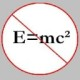You are currently browsing the tag archive for the ‘Mathematical Reflections’ tag.

In an earlier issue of Mathematical Reflections, Iurie Boreico (from Harvard) proposed the following problem.

Problem: A polynomial$p \in \mathbb{R}[X]$ is called a “mirror” if$|p(x)| = |p(-x)|$ . Let$f \in \mathbb{R}[X]$ and consider polynomials$p, q \in \mathbb{R}[X]$ such that$p(x) - p'(x) = f(x)$, and$q(x) + q'(x) = f(x)$. Prove that$p + q$ is a mirror iff$f$ is a mirror.

Two solutions – the pdf file size is around 1 Mb – to the above problem were proposed, and the one by the author is very close to the one I had worked out myself (partially) earlier but never really got around finishing it. So, I will post my solution here but at a slightly later time. In the meantime, you might be interested in finding a solution yourself.

Here is a list of problems (along with the names of the proposers) from Issue 1, 2008 of Mathematical Reflections that I like. The problem section actually contains 24 problems but I will post just a few here.$(1)$ The zeroes of the polynomial$P(x) = x^3 + x^2 + ax + b$ are all real and negative. Prove that$4a-9b \le 1$.

(Titu Andreescu)$(2)$ Let$x, y$ and$z$ be complex numbers such that$(y+z)(x-y)(x-z) = (z+x)(y-z)(y-x) = (x+y)(z-x)(z-y) = 1$$= 1$.

Determine all possible values of$(x+y)(y+z)(z+x)$.

(Alex Anderson)$(3)$ Prove that there is no polynomial$P \in \mathbb{R}[X]$ of degree$n \ge 1$ such that$P(x) \in \mathbb{Q}$ for all real$x$.

(Ivan Borsenco)$(4)$ Let$P$ be a complex polynomial of degree$n > 2$, and let$A$ and$B$ be two complex matrices such that$AB \ne BA$ and$P(AB) = P(BA)$. Prove that$P(AB) = cI_2$ for some complex number$c$.

(Titu Andreescu and Dorin Andrica)$(5)$ Let$n = \prod_{i=1}^{k}p_i$ where$p_1, p_2, \ldots p_k$ are distinct odd primes. Prove that there is a$A \in M_n(\mathbb{Z})$ with$A^m = I_n$ iff the symmetric group$S_{n+k}$ has an element of order$m$.

(Jean-Charles Mathieux)

2008, Issue 1 of Mathematical Reflections is out! Check out the problem section.

### Blog Stats

• 349,162 hitsprof dr drd horia or… on My first postprof drd horia orasa… on My first postprof dr mircea orasa… on Inequality with lognotedscholar on Self-referential Paradoxes, In…prof dr mircea orasa… on Inequality with logprof dr mircea orasa… on Inequality with logprof dr mircea orasa… on 2010 in reviewkenji on Basic category theory, Iprof dr mircea orasa… on Solution to POW-10: Another ha…prof drd horia orasa… on Continued fraction for eprof drd horia orasa… on Inequality with logprof dr mircea orasa… on Solution to POW-12: A graph co…prof dr mircea orasa… on The Character of Physical…prof dr mircea orasa… on Milk and Tea puzzleprof drd horia orasa… on Inequality with log